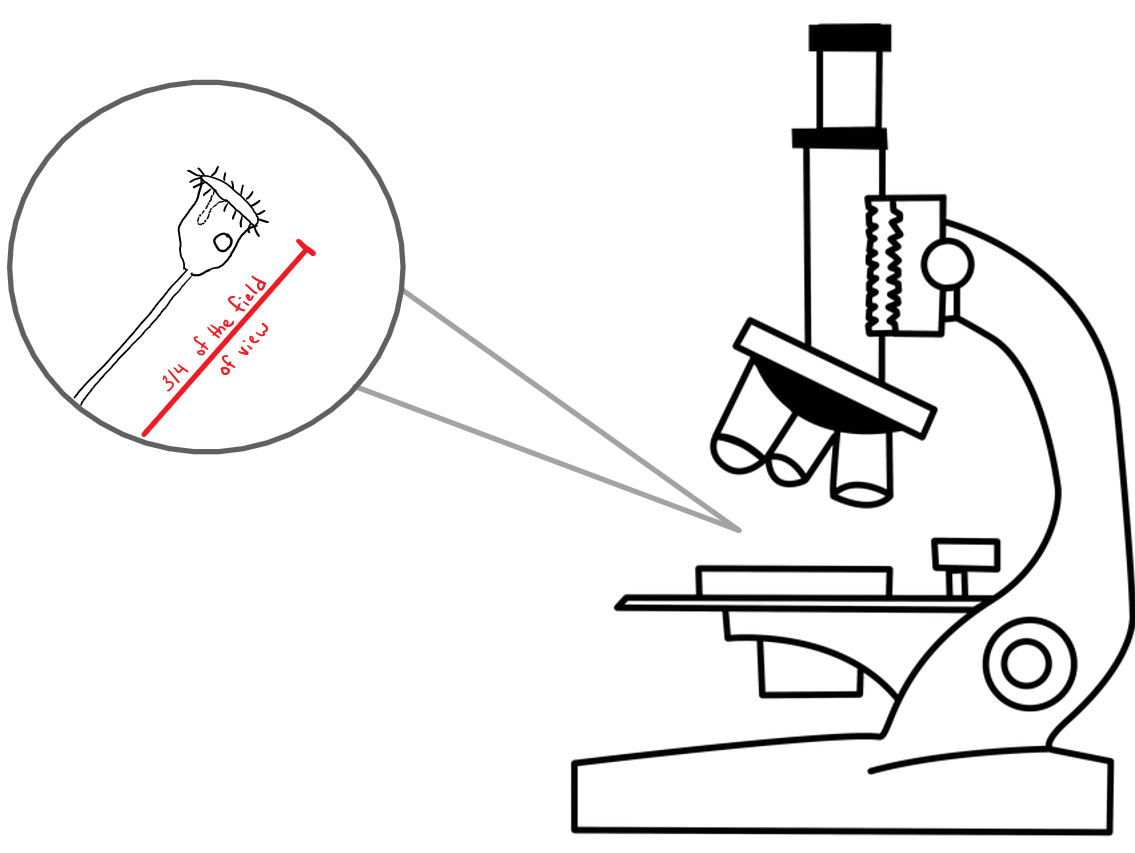## 17.2 Method 1: Estimation

### Field of View Diameter

First, determine the field of view diameter of your microscope for each objective setting.

These values might be provided to you in your lab manual. If so, proceed to the next section, estimating object size.

To calculate field of view diameter, we first need to know the field number (FN). When you look at your microscope eyepiece, the FN is displayed after the ocular lens magnification. For example, you might see 10x/16. In this case, 10x is the ocular lens magnification and 16 mm is the FN.

To calculate the field of view diameter use the following formula:

$d = \frac{f}{o}$

where

• $$d$$ = field of view diameter
• $$f$$ = field number
• $$o$$ = objective magnification

Assuming the FN on our microscope is 16 mm, let’s calculate the field of view diameter for the 4x objective.

$d = \frac{16 mm}{4} = 4 mm$

Repeat this process for the remainder of the objectives on your microscope. Your final result should look something like this:

Ocular-magnification Objective-magnification Total-magnification Field-of-view-diameter
10x 4x 40x 4 mm
10x 10x 100x 1.6 mm
10x 40x 400x 0.4 mm
10x 100x 1000x 0.16 mm

### Estimating Object Size

Next, estimate the size of the object you are looking at.

Since you know the diameter of the field of view for each lens, you can easily estimate the length or size of objects you are looking at by roughly comparing the object's size to the diameter of the circle. The formula for doing this is:

$o = fr \times d$ where

• $$o$$ = object size
• $$fr$$ = fraction of the field of view taken up by the object
• $$d$$ = field of view diameter

For example, suppose you are looking at a protozoan that extends 3/4 of the way across the field under 400x total magnification. Using the table above for the microscope in this example, we can see that at 400x total magnification, the objective magnification is 40x and the diameter of the field of view is 0.4 mm. Plugging these numbers into the formula above, we can estimate the length of the object at 0.3 mm.

$o = \frac{3}{4} \times 0.4mm = 0.3mm$Caption Estimating object size.
Image produced by Clerissa Copeland licensed under CC BY-NC-SA 4.0